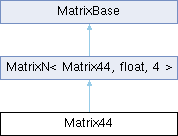MaterialX 1.38.7
Searching...
No Matches
Matrix44 Class Reference

A 4x4 matrix of floating-point values. More...

`#include <Types.h>`

Inheritance diagram for Matrix44:## Public Member Functions

Matrix44 (float m00, float m01, float m02, float m03, float m10, float m11, float m12, float m13, float m20, float m21, float m22, float m23, float m30, float m31, float m32, float m33)

Matrix Operations
Matrix44 getTranspose () const
Return the transpose of the matrix.

float getDeterminant () const
Return the determinant of the matrix.

Return the adjugate of the matrix.

Matrix44 getInverse () const
Return the inverse of the matrix.Public Member Functions inherited from MatrixN< Matrix44, float, 4 >
MatrixN (Uninit)

MatrixN (float s)

MatrixN (const float *begin, const float *end)

bool operator== (const Matrix44 &rhs) const
Return true if the given matrix is identical to this one.

bool operator!= (const Matrix44 &rhs) const
Return true if the given matrix differs from this one.

bool isEquivalent (const Matrix44 &rhs, float tolerance) const
Return true if the given matrix is equivalent to this one within a given floating-point tolerance.

RowArray & operator[] (size_t i)
Return the row array at the given index.

const RowArray & operator[] (size_t i) const
Return the const row array at the given index.

Matrix44 operator+ (const Matrix44 &rhs) const

MatrixNoperator+= (const Matrix44 &rhs)

Matrix44 operator- (const Matrix44 &rhs) const
Component-wise subtraction of two matrices.

MatrixNoperator-= (const Matrix44 &rhs)
Component-wise subtraction of two matrices.

Matrix44 operator* (float s) const
Component-wise multiplication of a matrix and a scalar.

MatrixNoperator*= (float s)
Component-wise multiplication of a matrix and a scalar.

Matrix44 operator/ (float s) const
Component-wise division of a matrix by a scalar.

MatrixNoperator/= (float s)
Component-wise division of a matrix by a scalar.

Matrix44 operator* (const Matrix44 &rhs) const
Compute the matrix product.

MatrixNoperator*= (const Matrix44 &rhs)
Compute the matrix product.

Matrix44 operator/ (const Matrix44 &rhs) const
Divide the first matrix by the second (computed as the product of the first matrix and the inverse of the second).

MatrixNoperator/= (const Matrix44 &rhs)
Divide the first matrix by the second (computed as the product of the first matrix and the inverse of the second).

Iterator begin ()

ConstIterator begin () const

Iterator end ()

ConstIterator end () const

float * data ()
Return a pointer to the underlying data array.

const float * data () const
Return a const pointer to the underlying data array.

## Static Public Attributes

static const Matrix44 IDENTITY

## Vector Transformations

Vector4 multiply (const Vector4 &v) const
Return the product of this matrix and a 4D vector.

Vector3 transformPoint (const Vector3 &v) const
Transform the given 3D point.

Vector3 transformVector (const Vector3 &v) const
Transform the given 3D direction vector.

Vector3 transformNormal (const Vector3 &v) const
Transform the given 3D normal vector.

static Matrix44 createTranslation (const Vector3 &v)
Create a translation matrix.

static Matrix44 createScale (const Vector3 &v)
Create a scale matrix.

static Matrix44 createRotationX (float angle)
Create a rotation matrix about the X-axis. More...

static Matrix44 createRotationY (float angle)
Create a rotation matrix about the Y-axis. More...

static Matrix44 createRotationZ (float angle)
Create a rotation matrix about the Z-axis. More...Public Types inherited from MatrixN< Matrix44, float, 4 >
using RowArray = typename std::array< float, N >

using Iterator = typename std::array< RowArray, N >::iterator

using ConstIterator = typename std::array< RowArray, N >::const_iteratorStatic Public Member Functions inherited from MatrixN< Matrix44, float, 4 >
static constexpr size_t numRows ()
Return the number of rows in this matrix.

static constexpr size_t numColumns ()
Return the number of columns in this matrix.Protected Attributes inherited from MatrixN< Matrix44, float, 4 >
std::array< RowArray, N > _arr

## Detailed Description

A 4x4 matrix of floating-point values.

Vector transformation methods follow the row-vector convention, with matrix-vector multiplication computed as v' = vM.

## ◆ createRotationX()

 Matrix44 createRotationX ( float angle )
static

Create a rotation matrix about the X-axis.

Parameters

## ◆ createRotationY()

 Matrix44 createRotationY ( float angle )
static

Create a rotation matrix about the Y-axis.

Parameters

## ◆ createRotationZ()

 Matrix44 createRotationZ ( float angle )
static

Create a rotation matrix about the Z-axis.

Parameters